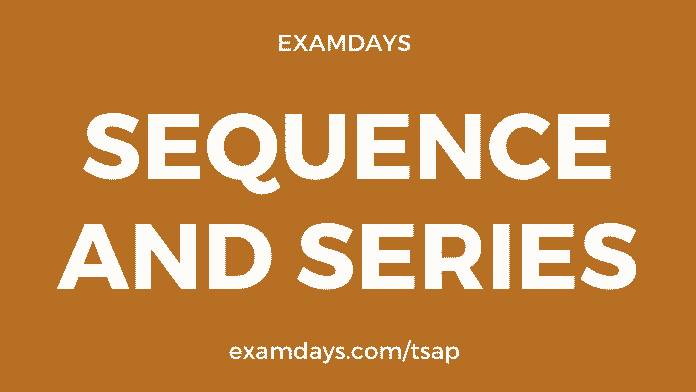0Sequence and Series pdf: Sequence and Series a topic of the Logical reasoning section, where 2 to 4 questions to be asked in every examination like banking, IBPS, SSC, RRB, State PSC, and other PSU examinations. These topics are very important for various Indian exams. Exam aspirants have to prepare for each and every topic of logical reasoning.

A series is a sequence of letters and numbers, which together gives a common rule, candidates have to find out their own rule based on the given question and follow that rule and get the final answer. In this series and sequence of questions, there is no definite rule or principle to solve the given questions.

We are posting Series and Sequence tricks to solve any questions, the rule should be made for candidates only, no specific rule or principle is available.

Series and Sequence rule has No rule and principle

There are divided into four categories

1. Number series
2. Letter Series
3. Missing Numbers
4. Repeated Series

Number Series

A number series is consists of various characteristics of numbers, and it has its own rules for solving particular questions, every question has a certain pattern or fixed pattern, we never expected a particular pattern, and candidates have to catch the pattern in order to solve the questions.

## Sequence and Series pdf

Example-1

Which number is replaced underlined mark in the given below series.?

2, 5, 10, 17, ____, 37?

A. 28

B. 27

C. 24

D. 26

Solutions

In these questions, the series is given 2,5,10,17,_,37. This means, this series has a certain pattern, where we have to find out the exam pattern in order to get the fixed answer.

By deep-looking pattern, the series code is increased by 2 and also difference by 2

5-2=3, 10-5 = 5, 17-10 = 7

so, we can conclude that, 7+2 = 9

=> 9 + 17 = 26.

Option: D is the right answer.

Example-2: 8, 15, 28, 53, _, 199

A. 103

B.105

C.101

D.102

Solution

The given series is 8, 15, 28, 53, _, 199

Observe the series and every number is multiplied by 2 and subtracted by 1

Which is x2-1, x2-2, x2-3, x2-4, x2-5, x2-6, x2-7, x2-8, and soon

53 x 2 -4 = 106 -4 = 102

Option: D is the right answer.

Letter Series

Letter series, the series which has alphabets were it from A to Z, In this case, candidates have to find the missing or next letter in the question by using English alphabetical tabular form. Also, the letters are available in both ascending and descending order in order to solve the questions.

Ascending Order

Descending Order

Example-1

ABX, EFT, IJP, MNI, ___?

A. QRH

B. QRI

C. QRS

D.OQT

Explanation

Follow the above ascending tabular for the letter sequence understanding purpose, Firts letter will be Q, proceeding like this answer is QRH

Option: A is he right answer.

Example-2

BXD, GSI, LNN, QIS, ____?

A. VDE

B. VDX

C.VWE

D.VEW

Solution

In order to find the right answer, we have to above alphabetic order form table for quick reference purposes.

As per the table, there is a gap between the first letter V, if repeated the same, the remaining two letters are D and X = VDX

option B is the right answer.

Missing Numbers

A missing number is a number, where it is skipped in the number series pattern, candidates have to trace the exam/approximate number in the given group of number series

Example: 636, 316, 156, 76,?

A. 26

B. 16

C. 36

D. 46

Solutions

The given number series is 636, 316, 156, 76, ?

Upon observation, the number series is decreasing half of it, hence, we will try to divide rule;

= 636 / 2 = 318 (we will apply -2 subtraction for verification) – 2 = 316

Next, we will repeat the same = 316/2 = 158-2 = 156 (seems to be, the pattern was correct)

Next; 156 / 2= 78 -2 = 76

76 / 2 = 38 -2 = 36

Hence, the option C is the right answer.

Repeated Series

The repeated series is like multiple missing numbers or number series or letter series, it any combination of missing numbers, candidates have to find the multiple missing numbers on given questions.

Example: aaa_bb_aab_baaa_bb

A. baab

B. bbaa

C. abab

D.babb

Solution

The given series is aaa_bb_aab_baaa_bb

In this series aaa is repeated 3 times, so that,

aaa | bbb | aaa |bbb | aaa | bbb

Hence, the correct answer is babb

Option D is the right answer.